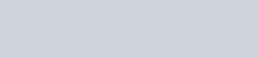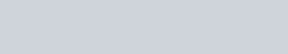NCERT Solutions for Class 9 Maths chapter 15 Exercise 15.1 – Probability
Hey, are you a class 9 Student and Looking for Ways to Download NCERT Solutions for Class 9 Maths chapter 15 Exercise 15.1? If Yes then you are at the right place.

Here we have listed Class 9 maths chapter 15 exercise 15.1 solutions in PDF that is prepared by Kota’s top IITian’s Faculties by keeping Simplicity in mind.

If you want to score high in your class 9 Maths Exam then it is very important for you to have a good knowledge of all the important topics, so to learn and practice those topics you can use eSaral NCERT Solutions.

In this article, we have listed NCERT Solutions for Class 9 Maths chapter 15 Exercise 15.1 that you can download to start your preparations anytime.

So, without wasting more time Let’s start.

### Download The PDF of NCERT Solutions for Class 9 Maths chapter 15 Exercise 15.1 “Probability”So, that’s all from this article. I hope you enjoyed this post. If you found this article helpful then please share it with other students.

#### All Questions of Chapter 15 Exercise 15.1

Once you complete the chapter 15 then you can revise Ex. 15.1 by solving following questions

Q1. In a cricket match, a batswoman hits a boundary 6 times out of 30 balls she plays. Find the probability that she did not hit a boundary.

Q2. 1500 families with 2 children were selected randomly, and the following data were recorded:Compute the probability of a family, chosen at random, having (i) 2 girls (ii) 1 girl (iii) No girl Also check whether the sum of these probabilities is 1.

Q3. Refer to Example 5, Section 14.4, Chapter 14 of NCERT. Find the probability that a student of the class was born in August. The statement of the data in the example is stated as below : In a particular section of class IX, 40 students were asked about the months of their birth and the following graph was prepared to represent the data:Q4. Three coins are tossed simultaneously 200 times with the following frequencies of different outcomes:If the three coins are simultaneously tossed again, compute the probability of 2 heads coming up.

Q5. An organization selected 2400 families at random and surveyed them to determine a relationship between income level and the number of vehicles in a family. The information gathered is listed in the table below:Suppose a family is chosen. Find the probability that the family chosen is
(i) earning Rs. 10000 – 13000 per month and owning exactly 2 vehicles.
(ii) earning Rs. 16000 or more per month and owning exactly 1 vehicle.
(iii) earning less than Rs. 7000 per month and does not own any vehicle.
(iv) earning Rs. 13000 – 16000 per month and owning more than 2 vehicles.
(v) owning not more than 1 vehicle.

Q6. Refer to chapter 14 (NCERT), the table below:(i) Find the probability that a student obtained less than 20% in the mathematics test.
(ii) Find the probability that a student obtained marks 60 or above.

Q7. To know the opinion of the student about the subject statistics, a survey of 200 students was conducted.
The data is recorded in the following table.Find the probability that a student chosen at random
(i) likes statistics,
(ii) does not like it.

Q8. Refer to Q.2, Exercise $14.2$ (NCERT). What is the empirical probability that an engineer life?
(i) less than $7 \mathrm{~km}$ from her place of work?
(ii) more than or equal to $7 \mathrm{~km}$ from her place of work?
(iii) within $\frac {1}{2} \mathrm{~km}$ from her place of work?

Q9. Activity: Note the frequency of two-wheelers, three-wheelers and four-wheelers going past during a time, interval, in front of your school gate. Find the probability that any one vehicle out of the total vehicles you have observed is a two-wheeler.

Q10. Activity: Ask all the students in your class to write a 3-digit number. Choose any student from the room at random. What is the probability that the number written by her/him is divisible by 3? Remember that a number is divisible by 3, if the sum of its digits is divisible by 3.

Q11. Eleven bags of wheat flour, each marked $5 \mathrm{~kg}$, actually contained the following weights of flour (in kg):
$\begin{array}{ccccccccccc}4 \cdot 97 & 5 \cdot 05 & 5 \cdot 08 & 5 \cdot 03 & 5 \cdot 00 & 5 \cdot 06 & 5 \cdot 08 & 4 \cdot 98 & 5 \cdot 04 & 5 \cdot 07 & 5 \cdot 00\end{array}$
Find the probability that any of these bags chosen at random contains more than $5 \mathrm{~kg}$ of flour.

Q12. In Q. 5, Exercise $14.2$ (NCERT), you were asked to prepare a frequency distribution table, regarding the concentration of sulphur dioxide in the air in parts per million of a certain city for 30 days. Using this table, find the probability of the concentration of sulphur dioxide in the interval $0 \cdot 12-0 \cdot 16$ on any of these days.

Q13. In Q.1, Exercise $14.2$ (NCERT), you were asked to prepare a frequency distribution table regarding the blood groups of 30 students of a class. Use this table to determine the probability that a student of this class, selected at random, has blood group $\mathrm{AB}$.

Download NCERT Class 9 Maths Book PDF

Download NCERT Class 9 Maths Exemplar PDF

Download Complete Solutions for Class 9 Maths chapter 15 PDF

Download Class 9 Maths Chapter 14 Exercise 14.1 Solutions PDF

Download Class 9 Maths Chapter 14 Exercise 14.2 Solutions PDF

Download Class 9 Maths Chapter 14 Exercise 14.3 Solutions PDF

Download Class 9 Maths Chapter 14 Exercise 14.4 Solutions PDF

If you have any Confusion related to NCERT Solutions for Class 9 Maths chapter 15 Exercise 15.1 then feel free to ask in the comments section down below.

To watch Free Learning Videos on Class 9 by Kota’s top Faculties Install the eSaral App